#Function Repository Resource:

# TriangleEdgeAngles

Given edge lengths of a triangle, return the angles opposite those edges

Contributed by: Wolfram Staff
 ResourceFunction["TriangleEdgeAngles"][{a,b,c}] returns the angles opposite edges (a,b,c).

## Examples

### Basic Examples

Edges of a 30-60-90 triangle:

 In:=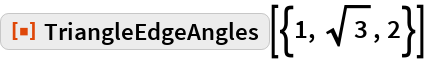Out=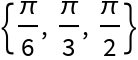### Scope

Edges for a 45-60-75 look complicated:

 In:=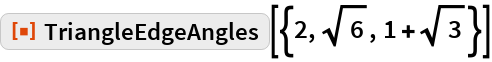Out=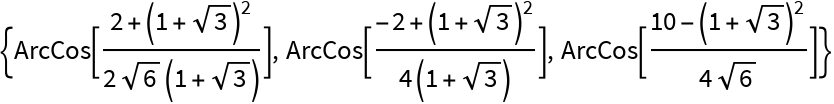RootReduce can help:

 In:=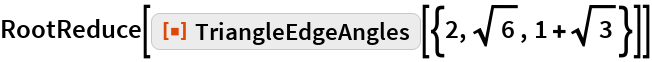Out=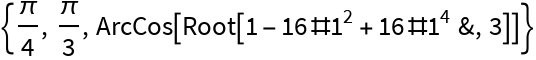In:=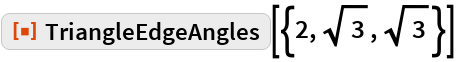Out=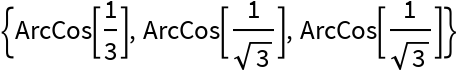## Requirements

Wolfram Language 11.3 (March 2018) or above